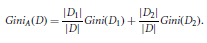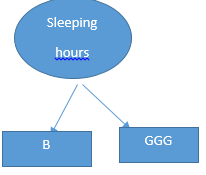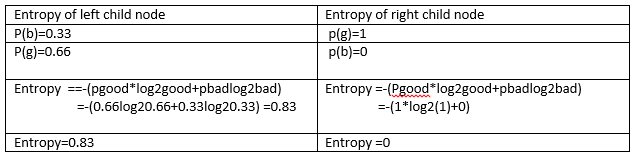Search

# DECISION TREE

In each and every moment in our life, we need to find the solutions for our problems. We might come across one or more solutions for a single problem. Out of which we need to decide the best fit. Decision tree is one of the prominent, simple algorithm to implement and easy to interpret. Decision tree belongs to supervised learning algorithm and used to solve the Regression and classification problems(CART). It is a two step process Learning and Classification step. Learning steps are used to develop model according to training data. Classification steps are used to test the model according to responses data.

Decision tree is flow-chart like graph where each node denotes a test of attribute value. Each branch represents the outcome of the test, leaf node represents the classes. Internal nodes has been divide into two or more classes.Above structure is a simple representation of decision tree .

ATTRIBUTE SELECTION

An attribute selection measure is a heuristic for selecting the splitting criteria. The attribute which having the best score has been chosen as the splitting attribute. There are three popular attribute selection measures metric

Information Gain:

This measure is based on attribute value. Let node N represent or hold the partition Node. The attribute with the highest information gain is chosen as the splitting attribute for node N. This attribute minimizes the information needed to classify the node resulting partition and reflects in random values or impurities in Node.

FORMULA 1:FORMULA 2:FORMULA 3:pi-pi is the probability that a Node in D belongs to class C(parent node)

D1,D2-represent the set of child node.

GINI INDEX

GINI INDEX or Gini impurity measures the degree or probability of a particular variable being wrongly classified when it is randomly chosen .if the all element belongs to single class its called "Pure". The attribute that maximizes the reduction in impurity (or has the

minimum Gini index) is selected as the splitting attribute.

FORMULA 4:FORMULA 5:pi-pi is the probability that a Node in D belongs to class C(parent node)

D1,D2-represent the set of child node.

GAIN RATIO:

The gain measure is biased toward the tests with many outcomes. It prefers to select attributes having a large number of values. The attribute with the maximum gain ratio is selected as the splitting attribute. However, if the split information approaches 0, the ratio becomes unstable.

In below illustrationHere we analyze the sleep duration which is crucial for the kids growth.

1.SLEEPING HOURS has been specified as GOOD(sleep > 8 hours) or BAD(sleep <8 hours/disturbed sleep).

2.PLAY has been specified as INDOOR or OUTDOOR

3.FOOD has been specified as LESS (unhealthy/less amount )or NORMAL(Healthy)

4.NIGHT BATH has been specified as YES or NO

Let us calculate information gain and Gini index for each attribute .

In the above table. Sleeping hours is parent node.

Sleeping Hours - Parent NodeEntropy for parent node = - ∑ P(value) *log2 (value)(using formula 1)

=-(0.75*log20.75+0.25*log20.25)

=-(075*(-0.41)+0.25*(-2)=-(-0.8)=0.8

I have chosen as FOODLess (BG) Normal(GG)Using Formula 1 we found result for Entropy of food(less) in left side and Entropy of Food(normal) in right side.

Using Formula 2 we need to calculate weight of average

Weight of average =(2/4*1+2/4*0)=0.5

Using Formula 3

Information gain=entropy parent-entropy(food, sleep)= 0.8 - 0.5= 0.33

Information Gain(Food)= 0.33

Internal node is NIGHT BATHY(BGG) N(G)Using Formula1 we found result for Entropy of Night bath(Y)(left) and Entropy of Night bath(n)(right).

Using Formula 2 we need to calculate the Weight of average for Night bath Node.

Weight of average =(3/4*0.83+1/4*0)=0.62

Using Formula 3:

Information gain=entropy parent-entropy(bath, sleep)=0.8-0.62=0.28

Information Gain(Night bath) 0.28OUTDOOR(GGG) INDOOR(B)Using Formula1 we found result for Entropy of Play(OUT)(left side) and Entropy of PLAY(IN)(right side).

Using Formula 2 we need to calculate the Weight of average for PLAY.

Weight of average =(3/4*0+1/4*0)=0

Using Formula 3:

Information gain = entropy parent - entropy(play ,sleep)= 0.8 - 0 = 0.8

Information Gain 0.8

As per calculation above Information Gain value for play is 0.8, Night bath is 0.28,Food is 0.33,In information Gain the highest value is taken to splitting the attributes for the class . In our cases

highest Information Gain is PLAY 0.8.

In the same scenario we going to calculate the GINI Index .

GINI Index for PLAY

P(out)=3/4=0.75

P(in)=1/4=0.25

If (kid play outdoor and sleep is good) =1

If (kid play indoor and sleep is bad) =0

If (kid play outdoor and sleep is good)=0

If (kid play outdoor and sleep is bad)=1

By using Gini Formula4:

Gini index(out)=1-(1*1+0*0)=1-1=0

Gini index(in)=1-(1*1+0)=0

By using Gini Formula 5:

Gini index(play)=0.75*0+0.25*0=0

Gini index(play)=0

GINI Index for Night Bath

P(y)=3/4=0.75

P(n)=1/4=0.25

If (kid takes bath and sleep is good) =2/3

If (kid takes bath and sleep is bad) =1/3

If (kid doesn’t bath and sleep is good)=1

If (kid doesn’t bath normal and sleep is bad)=0

By Using formula 4:

Gini index(yes)=1-(0.33*0.33+0.66*0.66)=1-(0.11+0.44)=0.45

Gini index(no)=1-(1*1+0)=0

By using formula 5:

Gini index(bath)=0.75*0.45+0.25*0=0.33

Gini index(Night Bath) = 0.33

P(less)=2/4 = 0.5

P(normal)=2/4 = 0.5

If (kid eat less and sleep is good) =0.5

If (kid eat less and sleep is bad) =0.5

If (kid eat normal and sleep is good)=1

If (kid eat normal and sleep is bad)=0

By using Formula 4:

Gini index(less)=1-(0.5*0.5+0.5*0.5)=1-(0.5)=0.5

Gini index(normal)=1-(1*1+0)=0

By using Formula 5:

Gini index(food)=0.5*0.5+0.5*0=0.25

Gini index(FOOD)=0.25

Therefore

Gini Index(play) = 0

Gini Index(Night Bath)=0.33

Gini Index(food)= 0.25

The attribute that maximizes the reduction in impurity or it has the minimum Gini index is selected as the splitting attribute. In the scenario we have PLAY has least Gini index is 0

IN the above illustration Information Gain for 0.8 and Gini index is 0 for Play which to connected to sleeping hours of Kid.

It is widely recognized that sleep is important for kids' health and well-being and that short sleep duration is associated with a wide range of negative health outcomes.

1. Decision tree like IF_ELSE statements which are simple to understand and easy to interpret .

2. Decision tree can automatically handle the missing data values.

3. No more feather scaling required in decision tree

4. Decision tree can handle both Numerical and categorical variables.

1. The major drawback of decision tree is overfitting of data which generally leads to creating a new node eventually tree became complex to interpret and it will give wrong prediction.

2. Not suitable for large dataset -eventually decision tree leads to overfitting .In the case we need to choose Random forest algorithm

3. Adding a new data point can lead to re-generation of the overall tree and all nodes need to be recalculated and recreated.

HAPPY LEARNING!